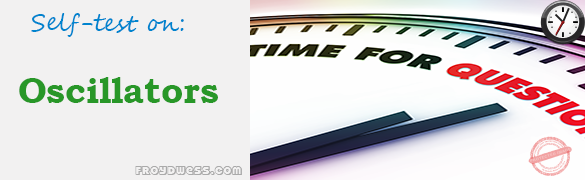# Floyd Self-test in Oscillators

(Last Updated On: December 8, 2017)This is the Self-test in Chapter 16: Oscillators from the book Electronic Devices Conventional Current Version, 9th edition by Thomas L. Floyd. If you are looking for a reviewer in Electronics Engineering this will definitely help you before taking the Board Exam.

### Floyd Self-test Chapter 16 Topic Outline

• Floyd Self-test in The Oscillator
• Floyd Self-test in Feedback Oscillators
• Floyd Self-test in Oscillators with RC Feedback Circuits
• Floyd Self-test in Oscillators with LC Feedback Circuits
• Floyd Self-test in Relaxation Oscillators
• Floyd Self-test in The 555 Timer as an Oscillator

### Start Practice Exam Test Questions

Choose the letter of the best answer in each questions.

1. An oscillator differs from an amplifier because the oscillator

• (a) has more gain
• (b) requires no input signal
• (c) requires no dc supply
• (d) always has the same output

2. One condition for oscillation is

• (a) a phase shift around the feedback loop of 180°
• (b) a gain around the feedback loop of one-third
• (c) a phase shift around the feedback loop of 0°
• (d) a gain around the feedback loop of less than 1

3. A second condition for oscillation is

• (a) no gain around the feedback loop
• (b) a gain of 1 around the feedback loop
• (c) the attenuation of the feedback circuit must be one-third
• (d) the feedback circuit must be capacitive

4. In a certain oscillator, The attenuation of the feedback circuit must be

• (a) 1
• (b) 0.01
• (c) 10
• (d) 0.02

5. For an oscillator to properly start, the gain around the feedback loop must initially be

• (a) 1
• (b) less than 1
• (c) greater than 1
• (d) equal to B

6. Wien-bridge oscillators are based on

• (a) positive feedback
• (b) negative feedback
• (c) the piezoelectric effect
• (d) high gain

7. In a Wien-bridge oscillator, if the resistances in the positive feedback circuit are decreased, the frequency

• (a) decreases
• (b) increases
• (c) remains the same

8. The Wien-bridge oscillator’s positive feedback circuit is

• (a) an RL circuit
• (b) an LC circuit
• (c) a voltage divider
• (d) a lead-lag circuit

9. A phase-shift oscillator has

• (a) three RC circuits
• (b) three LC circuits
• (c) a T-type circuit
• (d) a -type circuit

10. Colpitts, Clapp, and Hartley are names that refer to

• (a) types of RC oscillators
• (b) inventors of the transistor
• (c) types of LC oscillators
• (d) types of filters

11. The main feature of a crystal oscillator is

• (a) economy
• (b) reliability
• (c) stability
• (d) high frequency

12. An oscillator whose frequency is changed by a variable dc voltage is known as

• (a) a crystal oscillator
• (b) a VCO
• (c) an Armstrong oscillator
• (d) a piezoelectric device

13. The operation of a relaxation oscillator is based on

• (a) the charging and discharging of a capacitor
• (b) a highly selective resonant circuit
• (c) a very stable supply voltage
• (d) low power consumption

14. Which one of the following is not an input or output of the 555 timer?

• (a) Threshold
• (b) Control voltage
• (c) Clock
• (d) Trigger
• (e) Discharge
• (f ) Reset

Rate this: